# [PYTHON] Easy to try! A story about making a deep learning Othello and strengthening it until you beat yourself (Part 1)

It is said that Othello's AI algorithm was created by deep learning and became so strong that I could not win.

Also, in my case, it took about 2 months, but the implementation itself was not so difficult, so I would like to explain how to implement it.

What you will see in this article is how to create an Othello AI algorithm with deep learning. The basic idea is the same for other board games, so I think it can be diverted.

The target audience is those who have started using deep learning libraries such as TensorFlow and have been able to perform basic processing such as MNIST number classification, but do not know how to do it for other problems.

### Trigger

It was triggered by the fact that the Reversi Challenge was held at the Engineer and Life Community to which I belong. The rule of this contest was "anywhere you can stick to Reversi".

Just a little while ago, I was reading a book called "Deep Learning with Shogi AI", and I was thinking that I would like to make AI for board games someday, so I decided to enter. Did.

I started with the feeling that I would make an entry relatively easily, easily create a deep learning model and let AI hit it, but from the middle I gradually began to think "I want to beat myself" and improved. I was doing.

You may be wondering, "What about your Othello arm, even if you beat yourself?"

To be clear, it is weak.

As I played against deep learning, I got stronger little by little, but I'm generally thinking about this.

(My thoughts)

1. At first, it seems good to hit it so that it does not come out of the 4x4 square around the center
2. It is difficult to remove the squares on the edge of the board, so I want to remove them as much as possible.
3. The four corners can never be taken, so I want to take it anyway
4. Do not look ahead because it is troublesome

I used TensorFlow to create and train the model, but eventually it became an iOS app (not available on the App Store). If you clone it from GitHub, you can move it at hand. TokyoYoshida/reversi-charenge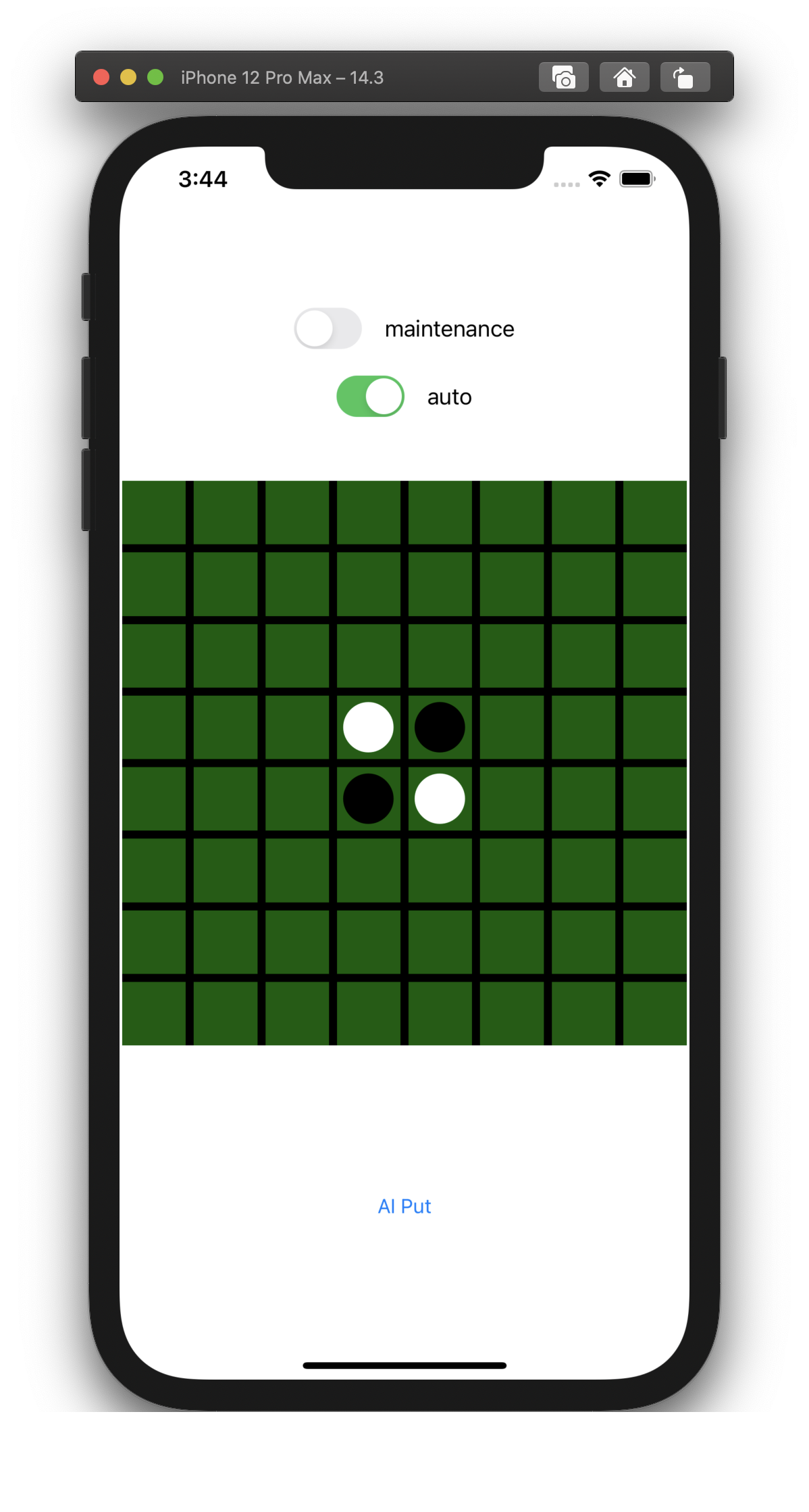As a function, you can play against AI, and you can freely create a board in maintenance mode. I haven't created a win / loss judgment function, so I need to count the number of my stones at the end.

The strength of AI is that AI wins about 80% against me. For some reason I sometimes win a complete victory with only the color of my stone. The reason is unknown, but it may be related to the fact that this AI doesn't look ahead and tends to catch up at the end without increasing its stones too much until the second half.

### Example of match result

I'm black and AI is white.

・ I was caught up at the end and it became almost pure white.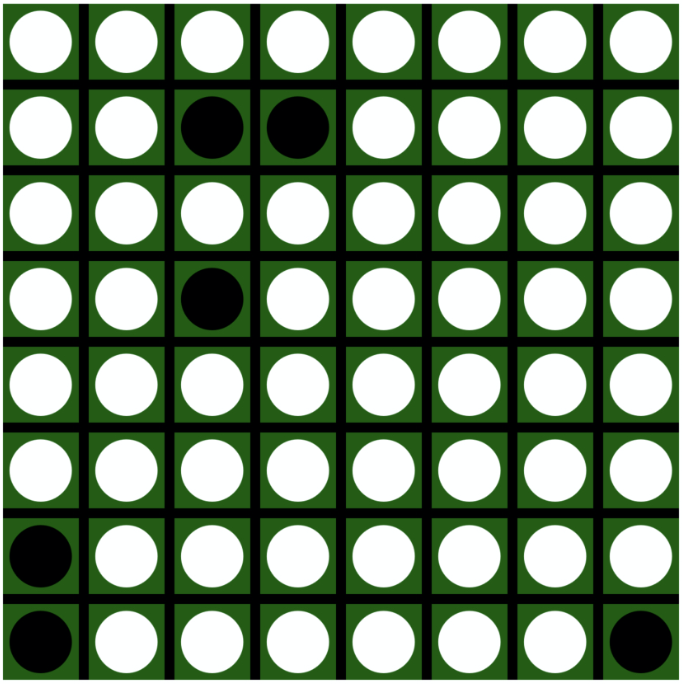・ I took three corners but lost. ..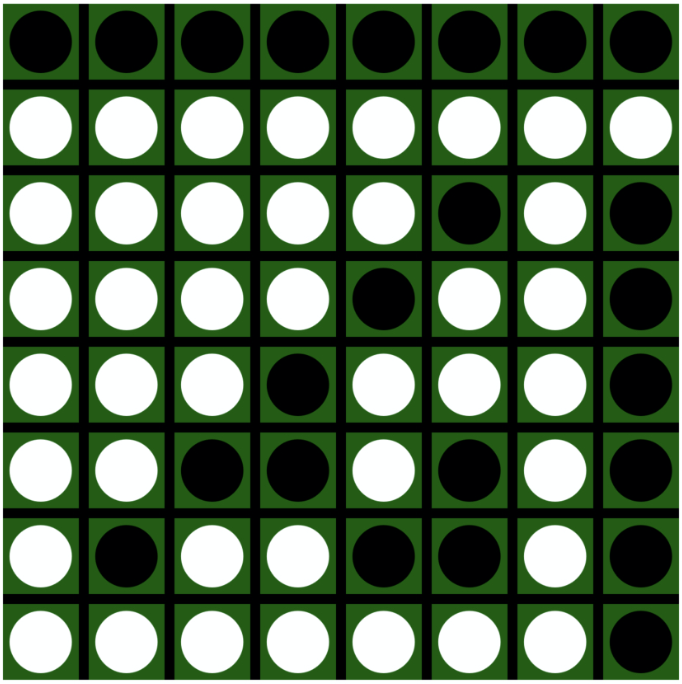During the match, I realized that even if I tried to take only the four corners, I couldn't always win, and if I had too many stones, I could only pass.

### Overview of how to make

I will go into the explanation of how to make it.

By passing the board data as an image, you can create an Othello AI by creating and training a neural network model that predicts the next move.

The "prediction of the next move" is a "classification problem that predicts which of the 64 squares is the best" because Othello has 8x8 squares.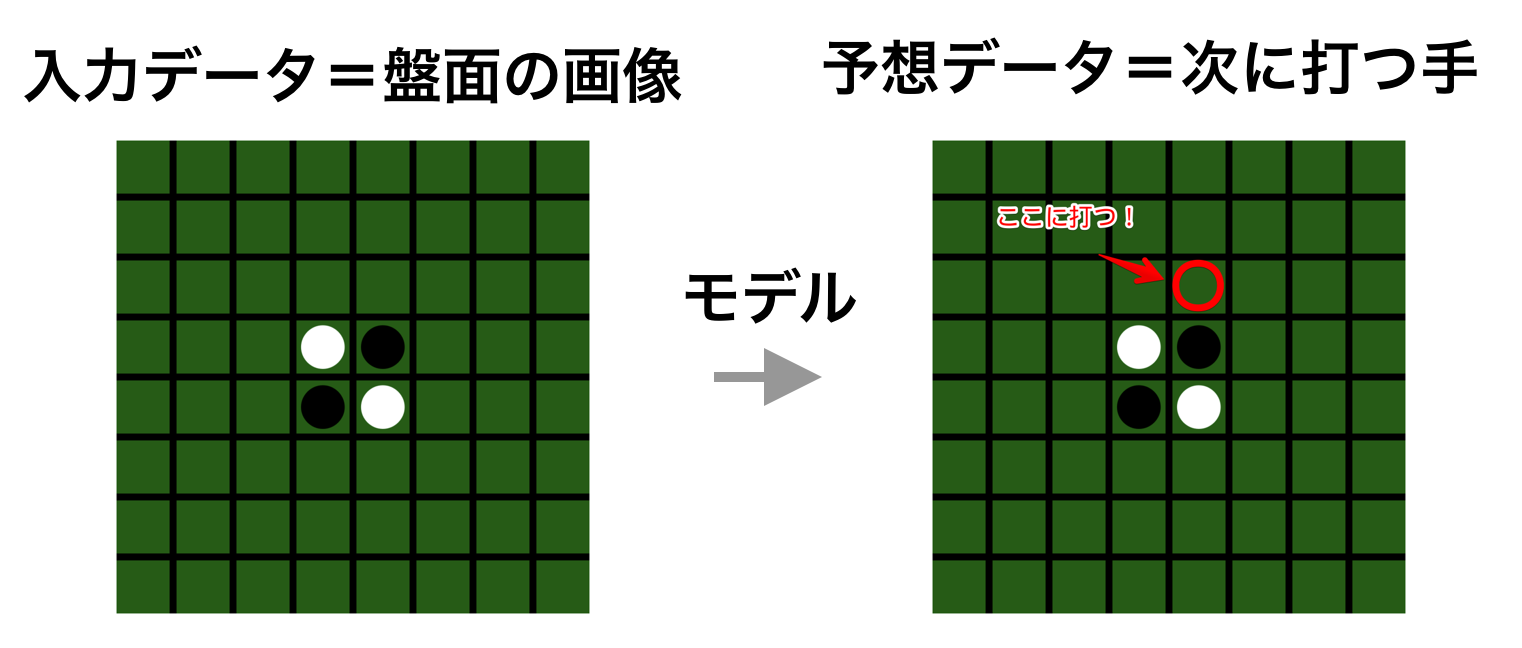** * An image is an array that expresses the board surface, not pixel data **

This imitates the mechanism of AlphaGo's SL policy network, and by using supervised learning to learn the image of the board from the game record data and the hand struck at that time to the neural network, when the board is given, the next move It is to be able to anticipate.

In the case of AlphaGo, we combined various methods, but this time we created only the SL policy network. As a bonus, I also made a combination of Monte Carlo tree search, which is a very different method from AlphaGo.

The procedure for making it is as follows.

1. Create learning data
2. Make a model
3. Train ---- (This explanation ends here) ----
4. Create UI (and search for Monte Carlo tree search)

Since this article is part 1, I will explain up to the point of training, but I will make up to the point where AI predicts Othello's hand.

Also, the implementation uses Python and runs on Google Colaboratory, but any environment where Python can run is fine.

### 1. Create learning data

This may be the hardest task. I will explain each one.

For the learning data, we will use the Othello game record database WTHOR published by the French Othello Federation, so download the data.

** ・ Convert game record data to csv **

Since the downloaded game record data is in binary format, convert it to csv with Conversion site. You can select the output format, but this time I used "All item output".

Reference: How to read Othello's game record database WTHOR (This is the article of the person who made this tool.)

After converting the game record data to csv, merge it into one file.

**-Decode csv data **

#### `NoteBook`

``````
import pandas as pd
import re
import numpy as np

df = pd.read_csv("wthor game record data.csv")

df = df.drop(index=df[df["transcript"].str.contains('transcript')].index)
``````

Of the read data, the column `transcript` is the actual game record information. You can see that the character strings are lined up, but one column value is the data for one game.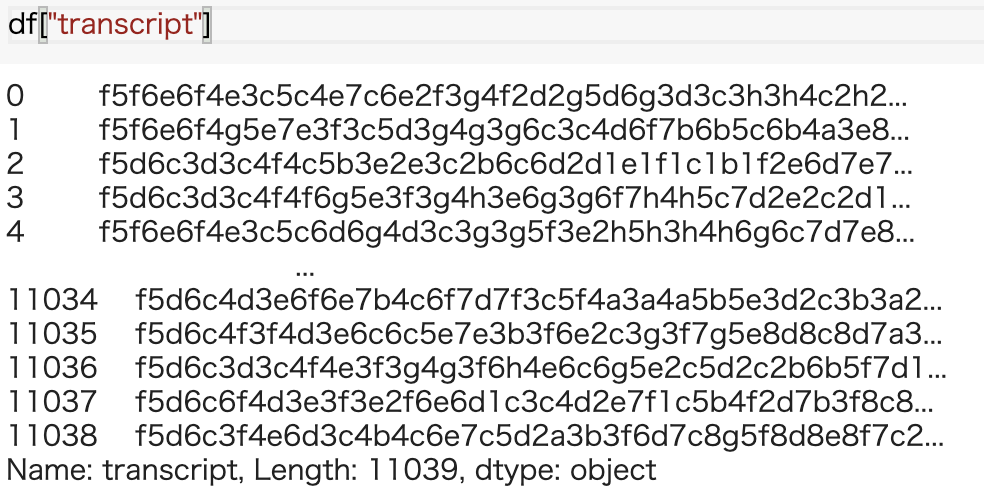The character string continues with `f5f6e6 ・ ・ ・`, but it is one move with two characters and is encoded as follows. 1st character ・ ・ ・ Column (a, b, c ・ ・ ・ h) 2nd character ・ ・ ・ Line (1,2,3 ・ ・ ・ 8) For example, `f5` means the 6th column from the left and the 5th row from the top.

It is difficult to understand that the `transcript` contains all the data of one game as it is, so Expand so that 1 line = 1 move. At the same time, the character string is decoded and converted into a column number and a row number.

#### `NoteBook`

``````

#Cut out two characters at a time using a regular expression
transcripts_raw = df["transcript"].str.extractall('(..)')

#Reorganize the Index into a table with 1 row and 1 move
transcripts_df = transcripts_raw.reset_index().rename(columns={"level_0":"tournamentId" , "match":"move_no", 0:"move_str"})

#Create a dictionary that converts column values ​​to numbers
def left_build_conv_table():
left_table = ["a","b","c","d","e","f","g","h"]
left_conv_table = {}
n = 1

for t in left_table:
left_conv_table[t] = n
n = n + 1

return left_conv_table

left_conv_table = left_build_conv_table()

#Convert column values ​​to numbers using dictionary
def left_convert_colmn_str(col_str):
return left_conv_table[col_str]

#Convert one hand to a number
def convert_move(v):
l = left_convert_colmn_str(v[:1]) #Convert column values
r = int(v[1:]) #Convert row values
return np.array([l - 1, r - 1], dtype='int8')

transcripts_df["move"] = transcripts_df.apply(lambda x: convert_move(x["move_str"]), axis=1)
``````

The data looks like this.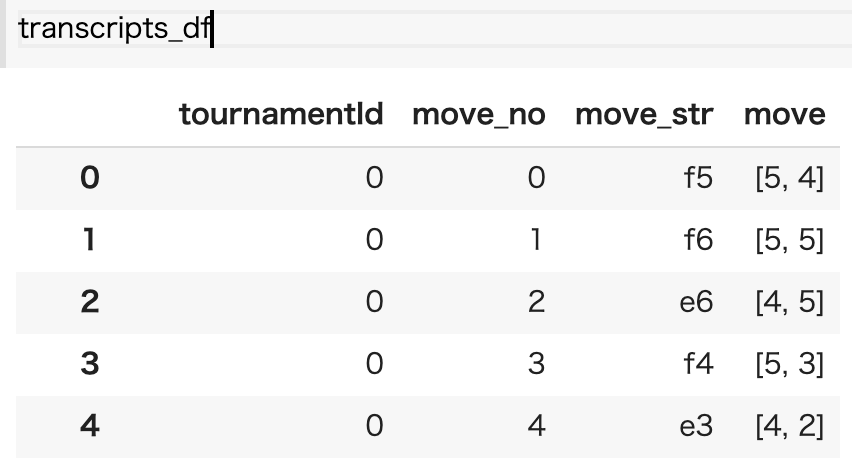** ・ Convert game record data to learning data **

The data of each move is expanded on the board and used as input data for learning.

The learning data is Othello's board as input data and one move at that time as teacher data. For example, if the board of Othello in the first example is used as training data, it will be as shown in the following figure.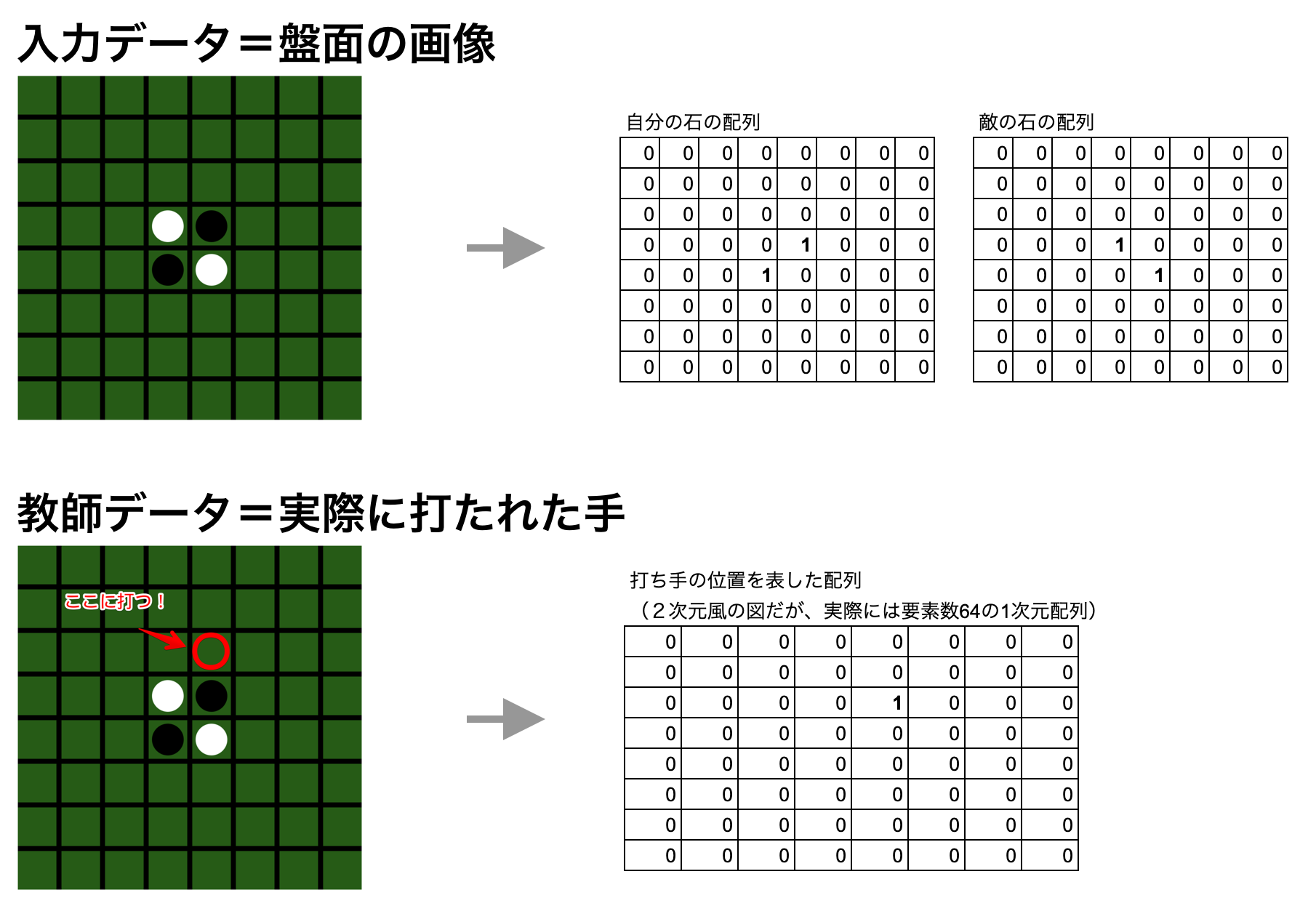The shapes are as follows. Training data = (number of batches, 2 (channel. Placement of own stones and placement of enemy stones), 8 (rows), 8 (columns)) Expected data = (Number of batches, 64 (0th in the upper left and 63rd in the lower right at the 8x8 board position))

Please note that the input data channels are not in the order of black → white, but in the order of your own stone → enemy stone. By doing this, both the data hit by black and the data hit by white can be used as learning data if they follow this arrangement.

In order to deploy on the board, if you make one move, you will put in a process to turn over the enemy's stone and record the board at that time.

In the case of a pass, the following data will also be in the hands of the same player. If you simply read the black number and the white number alternately, the board will be strange, so add the judgment process at the time of pass.

#### `NoteBook`

``````
#Check if it is on the board
def is_in_board(cur):
return cur >= 0 and cur <= 7

#One direction(Place a stone against direction) and flip the enemy stone if possible
def put_for_one_move(board_a, board_b, move_row, move_col, direction):
board_a[move_row][move_col] = 1

tmp_a = board_a.copy()
tmp_b = board_b.copy()
cur_row = move_row
cur_col = move_col

cur_row += direction
cur_col += direction
reverse_cnt = 0
while is_in_board(cur_row) and is_in_board(cur_col):
if tmp_b[cur_row][cur_col] == 1: #Invert
tmp_a[cur_row][cur_col] = 1
tmp_b[cur_row][cur_col] = 0
cur_row += direction
cur_col += direction
reverse_cnt += 1
elif tmp_a[cur_row][cur_col] == 1:
return tmp_a, tmp_b, reverse_cnt
else:
return board_a, board_b, reverse_cnt
return board_a, board_b, reverse_cnt

#Definition of direction (array elements correspond to ←, ↖, ↑, ➚, →, ➘, ↓, ↙)
directions = [[-1,0],[-1,1],[0,1],[1,1],[1,0],[1,-1],[0,-1],[-1,-1]]

#Place a stone in a certain position. Flip enemy stones if possible in all directions
def put(board_a, board_b ,move_row, move_col):
tmp_a = board_a.copy()
tmp_b = board_b.copy()
global directions
reverse_cnt_amount = 0
for d in directions:
board_a ,board_b, reverse_cnt = put_for_one_move(board_a, board_b, move_row, move_col, d)
reverse_cnt_amount += reverse_cnt

return board_a , board_b, reverse_cnt_amount

#Make sure there are no stones on the board
def is_none_state(board_a, board_b, cur_row, cur_col):
return board_a[cur_row][cur_col] == 0 and board_b[cur_row][cur_col] == 0

#Check if stones can be placed on the board (the rule is that stones can only be placed in positions where the enemy stones can be reversed)
def can_put(board_a, board_b, cur_row, cur_col):
copy_board_a = board_a.copy()
copy_board_b = board_b.copy()
_,  _, reverse_cnt_amount = put(copy_board_a, copy_board_b, cur_row, cur_col)
return reverse_cnt_amount > 0

#Check if the board needs to pass
def is_pass(is_black_turn, board_black, board_white):
if is_black_turn:
own = board_black
opponent = board_white
else:
own = board_white
opponent = board_black
for cur_row in range(8):
for cur_col in range(8):
if is_none_state(own, opponent, cur_row, cur_col) and can_put(own, opponent, cur_row, cur_col):
return False
return True

#Variable initialization
b_tournamentId = -1 #Tournament number
board_black = [] #Board condition for black (for saving one game)
board_white = [] #The state of the board for White (for saving one game)
boards_black = [] #Board condition for black (for preserving all tournaments)
boards_white = [] #Board condition for white (for preserving all tournaments)
moves_black = [] #Black hand (for storing all tournaments)
moves_white = [] #White hand (for storing all tournaments)
is_black_turn = True # True =Black turn, False=White turn
#Switch the turn (black turn or white turn)
def switch_turn(is_black_turn):
return is_black_turn == False #Switch turns

#A function that reads one game record data and creates learning data
def process_tournament(df):
global is_black_turn
global b_tournamentId
global boards_white
global boards_black
global board_white
global board_black
global moves_white
global moves_black
if df["tournamentId"] != b_tournamentId:
#Reset the board to the initial state when the tournament is switched
b_tournamentId = df["tournamentId"]
board_black = np.zeros(shape=(8,8), dtype='int8')
board_black = 1
board_black = 1
board_white = np.zeros(shape=(8,8), dtype='int8')
board_white = 1
board_white = 1
is_black_turn = True
else:
#Switch turns
is_black_turn = switch_turn(is_black_turn)
if is_pass(is_black_turn, board_black, board_white): #Check if it should pass
is_black_turn = switch_turn(is_black_turn) #Switch turns if you should pass

#If it is a black turn, save the state of the black board, if it is a white turn, save the state of the white board
if is_black_turn:
boards_black.append(np.array([board_black.copy(), board_white.copy()], dtype='int8'))
else:
boards_white.append(np.array([board_white.copy(), board_black.copy()], dtype='int8'))

#Get a move
move = df["move"]
move_one_hot = np.zeros(shape=(8,8), dtype='int8')
move_one_hot[move][move] = 1

#If it's black's turn, change the arrangement of yourself → enemy from black → white and set the move. If it's white's turn, set the move in the order of white → black
if is_black_turn:
moves_black.append(move_one_hot)
board_black, board_white, _ = put(board_black, board_white, move, move)
else:
moves_white.append(move_one_hot)
board_white, board_black, _ = put(board_white, board_black, move, move)

#Expand game record data into learning data
transcripts_df.apply(lambda x: process_tournament(x), axis= 1)
``````

When I run the above code, each variable looks like this: boards_black ・ ・ ・ The state of the board just before black makes a move boards_white ・ ・ ・ The state of the board just before White makes a move moves_black ・ ・ ・ Hands struck by black moves_white ・ ・ ・ Hands struck by white

Use these to create learning data. All you have to do is connect the black and white boards and the strikers into a single piece of data.

#### `NoteBook`

``````
x_train = np.concatenate([boards_black, boards_white])
y_train = np.concatenate([moves_black, moves_white])
#Since the teacher data is 8x8 2D data, reshape it into 64 element 1D data.
y_train_reshape = y_train.reshape(-1,64)
``````

I downloaded 20 years' worth of data from WTHOR and did this processing, and now I have 5.35 million board data. You should be able to create even stronger AI by combining data that has not been downloaded yet.

### 2. Make a model

Finally make a model.

The input data is an array of shape = (number of batches, 2,8,8) as explained above. This is Permute (transposed) at the beginning, but this is for the convenience of TensorFlow. I thought it would be okay to specify Channels First (batch, C, H, W) for Conv2D, but for some reason Conv2D did not accept Channels First, so I hurried to Channels Last (batch, H, W, C). I'm converting. (If I knew this, I should have prepared such data from the beginning ..)

Next, 12 convolution layers with kernel size 3, a convolution layer with kernel size 1, and finally a bias layer are inserted and applied to the SoftMax function. The result is an array of shape = (number of batches, 64). In the 64 elements, the probability of the next move is entered as a number from 0 to 1 for each square of Othello. The closer it is to 1, the better the hand.

Although it is a bias layer, tf.keras did not have a bias layer, so I made it as a Bias class.

#### `NoteBook`

``````
import tensorflow as tf
from tensorflow import keras
from tensorflow.keras import layers
from keras.engine.topology import Layer

class Bias(keras.layers.Layer):
def __init__(self, input_shape):
super(Bias, self).__init__()
self.W = tf.Variable(initial_value=tf.zeros(input_shape[1:]), trainable=True)

def call(self, inputs):
return inputs + self.W

model = keras.Sequential()
``````

Once you have a model, compile it.

The optimizer is the same SGD as AlphaGo, and the loss function is a classification problem, so use `categorical_crossentropy`.

#### `NoteBook`

``````
model.compile(keras.optimizers.SGD(lr=0.01, momentum=0.0, decay=0.0, nesterov=False), 'categorical_crossentropy', metrics=['accuracy'])
``````

### 3. Train

I will train.

The training was done on an instance of Google AI Platform because the timeout is severe with Google Colab. The trained model is saved even if it is interrupted in the middle by enclosing it with `try` ~` except`.

#### `NoteBook`

``````
try:
model.fit(x_train, y_train_reshape, epochs=600, batch_size=32, validation_split=0.2)
except KeyboardInterrupt:
model.save('saved_model_reversi/my_model')
print('Output saved')
``````

After learning about 6 hours and 4 epochs on an instance of AI Platform (NVIDIA Tesla T4 GPU), val_accuracy became 0.59, so I stopped learning there.

AplhaGo learned about 28.4 million boards and the accuracy was 57%.

### Difficulties in model training and countermeasures

At the beginning, I trained the model for 12 hours, but the accuracy was only about 0.25, and I was wondering what happened even if I actually played against it.

After investigating the cause, I found the following problem.

--There was a bug in the process of expanding the board from the game record data. For this reason, I was learning the wrong board --The method of specifying Permute of the model was incorrect. This trained weird images --After the Bias layer, I reshaped it to (8,8). 8 It had become a classification problem

Deep learning is hard to notice that there is a mistake, because even if you read meaningless data, it will somehow give an answer. Also, even if you extract and check the state in the middle of processing, it is difficult to judge whether it is correct or incorrect.

Therefore, we conducted an investigation using the following procedure.

** 1. Set criteria for deep learning output quality **

This time, the standard was to "win me." We have also set a reference value for Accuracy. The accuracy of 0.25 is lower than that of other Othello AIs, so I guessed from other cases and wanted it to exceed at least 0.35.

To put it the other way around, I wouldn't have investigated without such a standard, so I think I couldn't find the above problem.

** 2. Classify the parts that can cause model problems into MECE **

The major categories are data, models, and parameters. After arranging these in a tree shape, we will dig down the branches of the tree one by one.

If you replace the random data or implementation without being aware of this, you will not be able to narrow the scope of what is right and what is wrong.

** 3. Find the cause by subdividing the whole process from data processing to model output.

It's the same story as 2., but here we will focus on the data flow and subdivide it. For example, you may notice a problem if you try to enter a simple value such as all 0s in a neural network and check the output of a particular layer. (There were many things I didn't understand at all ...)

### Let AI predict the move

After learning, you are ready to predict what to do. Let's try and predict.

#### `NoteBook`

``````
#Gives the initial board data of Othello
board_data = np.array([[
[[0,0,0,0,0,0,0,0],
[0,0,0,0,0,0,0,0],
[0,0,0,0,0,0,0,0],
[0,0,0,0,1,0,0,0],
[0,0,0,1,0,0,0,0],
[0,0,0,0,0,0,0,0],
[0,0,0,0,0,0,0,0],
[0,0,0,0,0,0,0,0]],

[[0,0,0,0,0,0,0,0],
[0,0,0,0,0,0,0,0],
[0,0,0,0,0,0,0,0],
[0,0,0,1,0,0,0,0],
[0,0,0,0,1,0,0,0],
[0,0,0,0,0,0,0,0],
[0,0,0,0,0,0,0,0],
[0,0,0,0,0,0,0,0]]]],dtype=np.int8)

#Predict the move
model.predict(board_data)
#Output result
# array([[2.93197723e-11, 1.42428486e-10, 7.34781472e-11, 2.39318716e-08,
#         1.31301447e-09, 1.50736756e-08, 9.80145964e-10, 2.52176102e-09,
#         3.33402395e-09, 2.05685264e-08, 2.49693510e-09, 3.53782520e-12,
#         8.09815548e-10, 6.63711930e-08, 2.62752469e-08, 5.35828759e-09,
#         4.46924164e-10, 7.42555386e-08, 2.38477658e-11, 3.76452749e-06,
#         6.29236463e-12, 4.04659602e-07, 2.37438894e-06, 1.51068477e-10,
#         1.81150719e-11, 4.47054616e-10, 3.75479488e-07, 2.84151619e-14,
#         3.70454689e-09, 1.66316525e-07, 1.27947108e-09, 3.30583454e-08,
#         5.33877942e-10, 5.14411222e-11, 8.31681668e-11, 6.85821679e-13,
#         1.05046523e-08, 9.99991417e-01, 3.23126500e-07, 1.72151644e-07,
#         1.01420455e-10, 3.35642431e-10, 2.22305030e-12, 5.21605148e-10,
#         5.75579229e-08, 9.84997257e-08, 3.62535673e-07, 4.41284129e-08,
#         2.43385506e-10, 1.96498547e-11, 1.13820758e-11, 3.01558468e-14,
#         3.58017758e-08, 8.61415117e-09, 1.17988044e-07, 1.36784823e-08,
#         1.19627297e-09, 2.55619081e-10, 9.82019244e-10, 2.45560993e-12,
#         2.43100295e-09, 8.31343083e-09, 4.34338648e-10, 2.09913722e-08]],
#       dtype=float32)
``````

Since the output result is an array for each square, you can get the move by taking the maximum value.

#### `NoteBook`

``````
np.argmax(model.predict(board_data))

#Output result
# 37
``````

The 37th from the upper left seems to be the best move.

### Finally

Eventually, I would like to write Part 2.

The second is the operation on iOS devices. I will write about how to make deep learning work on iOS devices, and the story of trying to get the minimax method and Monte Carlo tree search and failing.

(I'm sorry if I didn't write it)

In addition, Note regularly publishes iOS development, especially CoreML, ARKit, Metal, etc. https://note.com/tokyoyoshida